Next: Capillary Curves Up: Surface Tension Previous: Angle of Contact

# Jurin's Law

Consider a situation in which a narrow, cylindrical, glass tube of radiusis dipped vertically into a liquid of density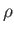, and the liquid level within the tube rises a height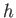above the free surface as a consequence of surface tension. (See Figure 3.3.) Suppose that the radius of the tube is much less than the capillary length. A tube for which this is the case is generally known as a capillary tube. According to the discussion in Section 3.4, the shape of the internal liquid/air interface within a capillary tube is not significantly affected by gravity. Thus, from Section 3.3, the interface is a segment of a sphere of radius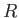(say). Ifis the angle of contact of interface with the glass then simple geometry (see Figure 3.3) reveals that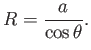(3.20)

Hence, from Equation (3.13), the mean curvature of the interface is given by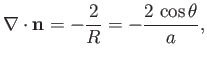(3.21)

where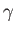is the associated surface tension. [The minus sign in the previous expression arises from the fact thatpoints towards the center of curvature of the interface, whereas the opposite is true for Equation (3.13).] Finally, from Equation (3.15), application of the Young-Laplace equation to the interface yields(3.22)

which can be rearranged to give(3.23)

This result, which relates the height,, to which a liquid rises in a capillary tube of radiusto the liquid's surface tension,, is known as Jurin's law, and is named after its discoverer, James Jurin (1684-1750). The assumption that the radius of the tube is much less than the capillary length is equivalent to the assumption that the height of the interface above the free surface of the liquid is much greater than the radius of the tube. This follows, from Equations (3.16) and (3.23), because(3.24)

Thus, the ordering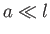implies that.

For the case of water at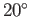, assuming a contact angle of, Jurin's law yields(Batchelor 2000). Thus, water rises a height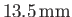in a capillary tube of radius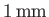, but rises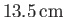in a capillary tube of radius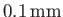. In the case of a liquid, such a mercury, that has an oblique angle of contact with glass, so that, the liquid level in a capillary tube is depressed below that of the free surface (i.e.,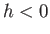).Next: Capillary Curves Up: Surface Tension Previous: Angle of Contact
Richard Fitzpatrick 2016-03-31